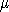# Spherical Shell using Gauss' Law

## Homework Statement

An insulator is in the shape of a spherical shell. The insulator is defined by an inner radius a = 4 cm and an outer radius b = 6 cm and carries a total charge of Q = + 9C (1C = 10-6 C). You may assume that the charge is distributed uniformly throughout the volume of the insulator.

What is Ey, the y-component of the electric field at point P which is located at (x,y) = (0, -5 cm)?

## Homework Equations

Φnet = Qenclosed / ∈0 = E × ∫surface dA

## The Attempt at a Solution

I found the charge density ρ:
Total charge 9e-6 C / ( 4/3 π (0.062 - 0.042)) = ρ

Then, with my Gaussian surface a sphere with radius 5 cm, I found Qenclosed
Qenclosed = ρV = ρ * 4/3 π (0.052 - 0.042)

I know that
Φnet = Qenclosed / ∈0 = E × ∫surface dA
which I can rearrange such that
E (this is what I need to find - the electric field on the surface) = Qenclosed / (ε0 * ∫surface dA)
And since ∫surface dA = 4 π r2
I can find E:
E = 1/4πε0 * Qenclosed / r2 = 1/4πε0 * 4.05e-6 / 0.052 = 14563800

This isn't correct, though. Where did I go wrong? I think it is in those last few equations - do I have the wrong area?

The units do work out: k has units N*m2/C2, Qenc has units C, and r2 is in m2, which works out to N/C

TSny
Homework Helper
Gold Member
Hello! Welcome to PF!

Your expression for the volume of a sphere is not correct. The power of r is not right.

Otherwise, your method looks good to me•kgleeso
Oops! Thank you.